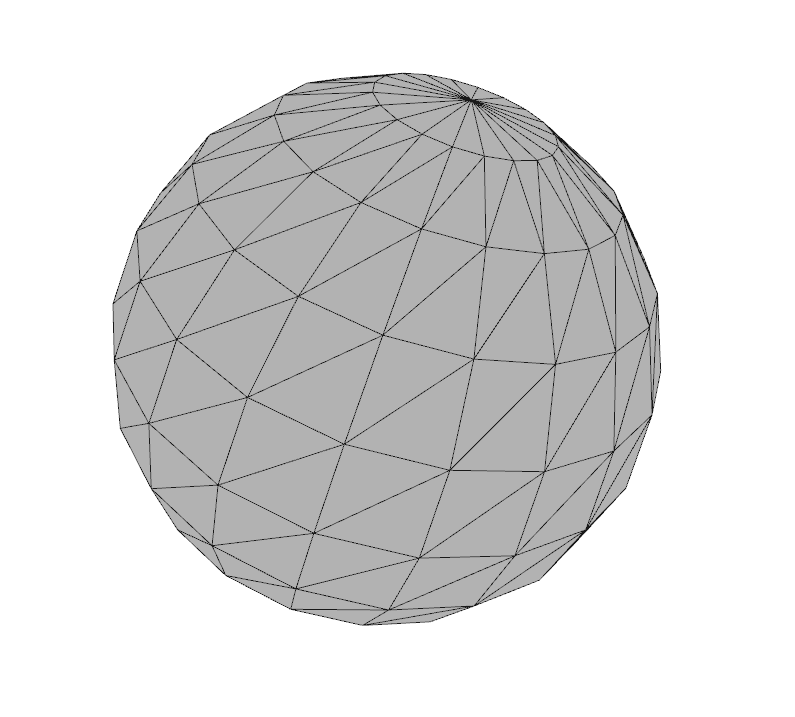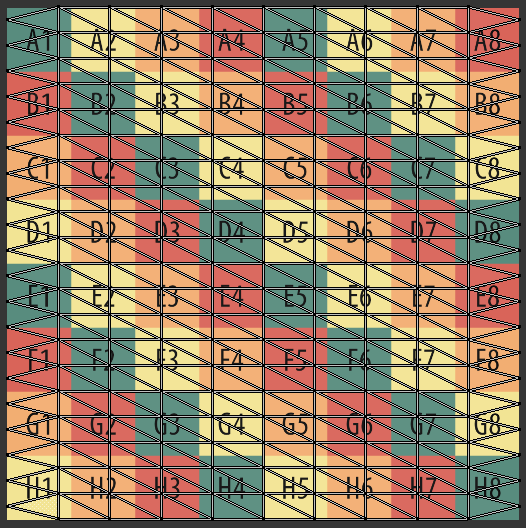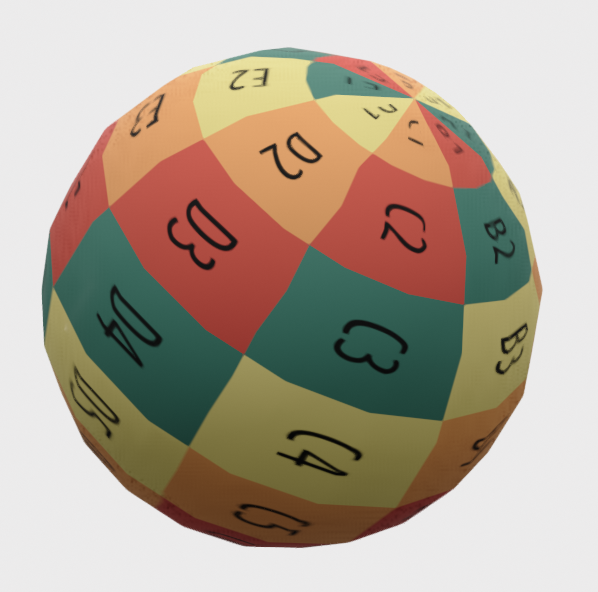# UV Maps#

## UV Maps#

### What is a UV Map ?#

[Ref: Wikipedia] UV mapping is the 3D modeling process of projecting a 2D image to a 3D model’s surface for texture mapping. The letters “U” and “V” denote the axes of the 2D texture because “X”, “Y”, and “Z” are already used to denote the axes of the 3D object in model space. UV texturing permits polygons that make up a 3D object to be painted with color (and other surface attributes) from an ordinary image. The image is called a UV texture map.

### How to add custom UV maps ?#

• UV Map coordinates `(U, V)` are stored as `std::vector<Eigen::Vector2d>` of length `3 x number of triangles`. So, there is a set of 3 (U, V) coordinates for each triangle, each associated with it’s vertices.

• One may assume the UV map, maps a texture image of height and width of length 1.0 to the geometry. So, the range of U and V is from 0.0 to 1.0 (both inclusive).

## Quick Reference to default UV Maps for some primitive shapes provided by Open3D#

The examples below all assume the following code preamble:

```import open3d as o3d
import open3d.visualization.rendering as rendering

material = rendering.MaterialRecord()
```

### Example Texture Map#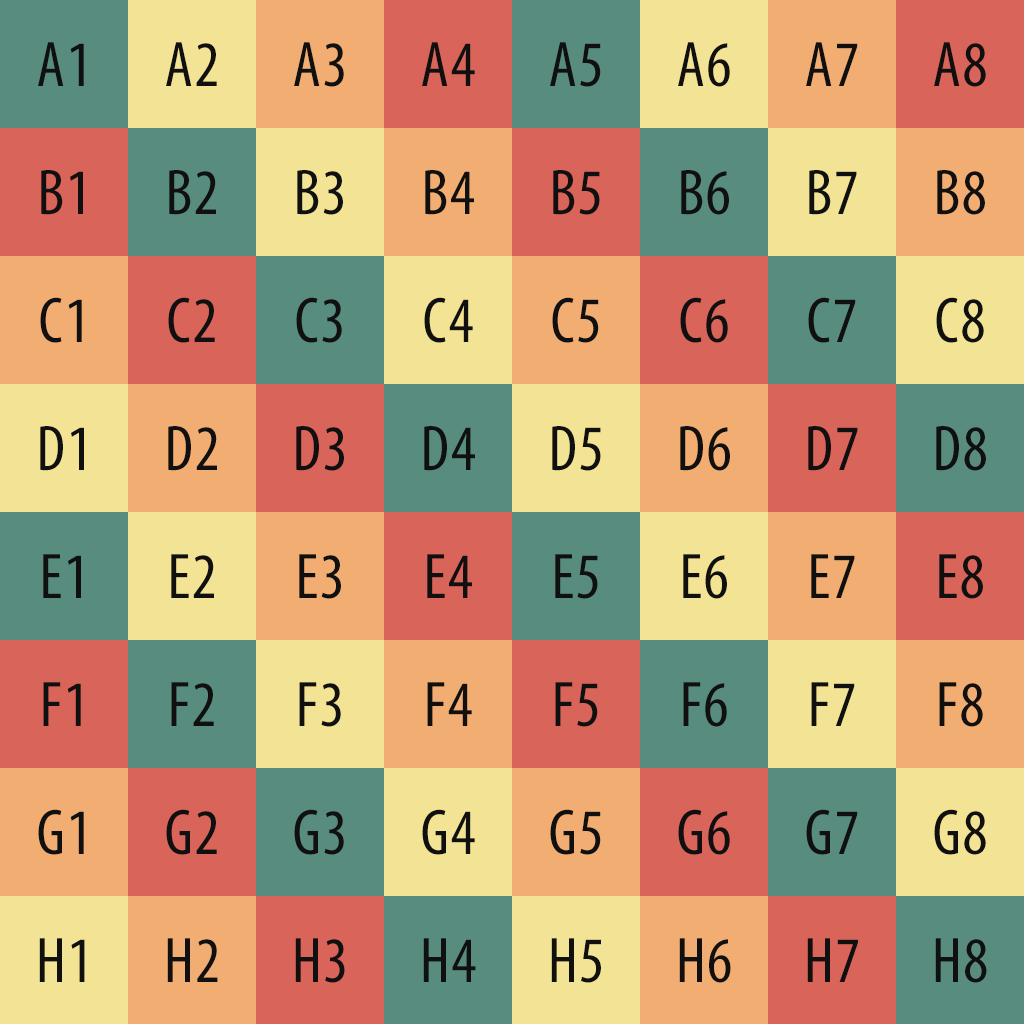### Box (map uv to each face = false)#

```box = o3d.geometry.TriangleMesh.create_box(create_uv_map=True)
o3d.visualization.draw({'name': 'box', 'geometry': box, 'material': material})
```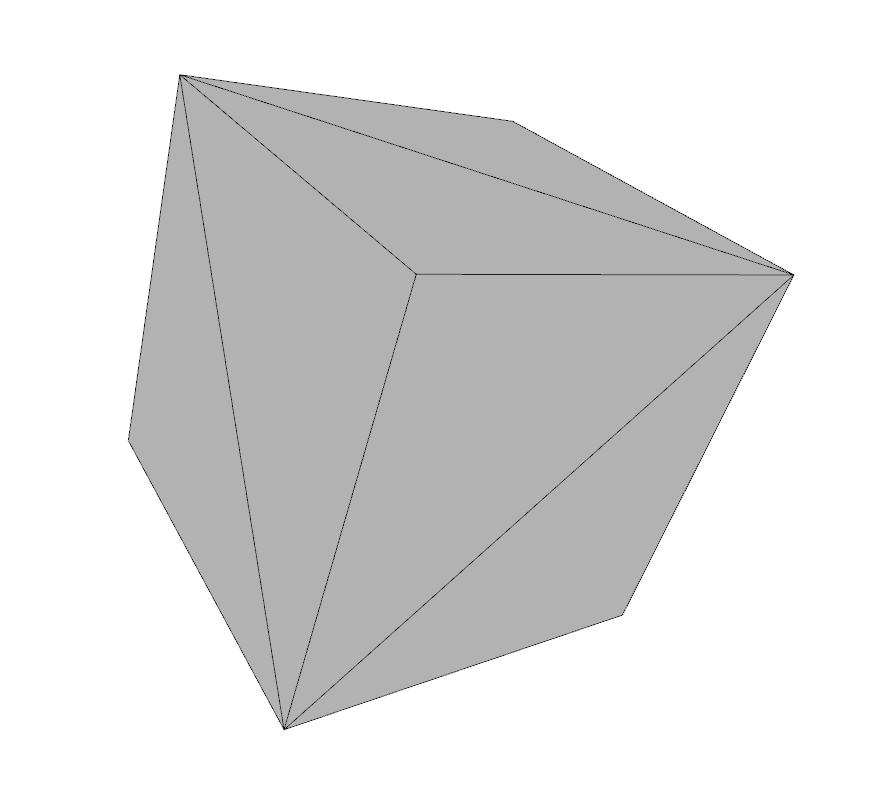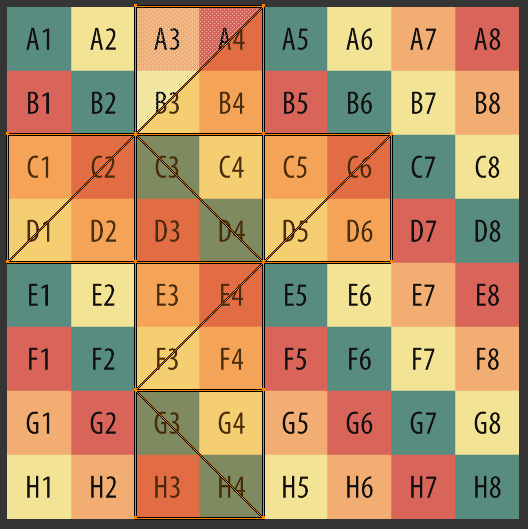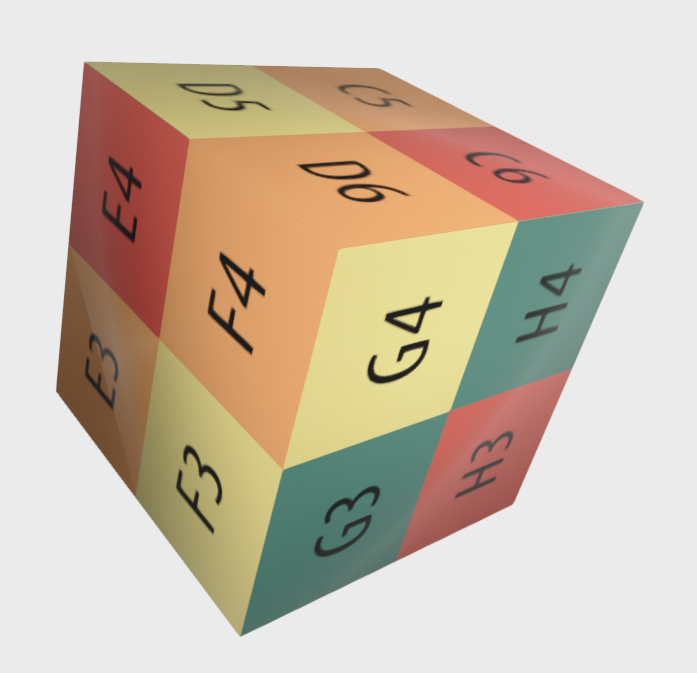### Box (map uv to each face = true)#

```box = o3d.geometry.TriangleMesh.create_box(create_uv_map=True, map_texture_to_each_face=True)
o3d.visualization.draw({'name': 'box', 'geometry': box, 'material': material})
```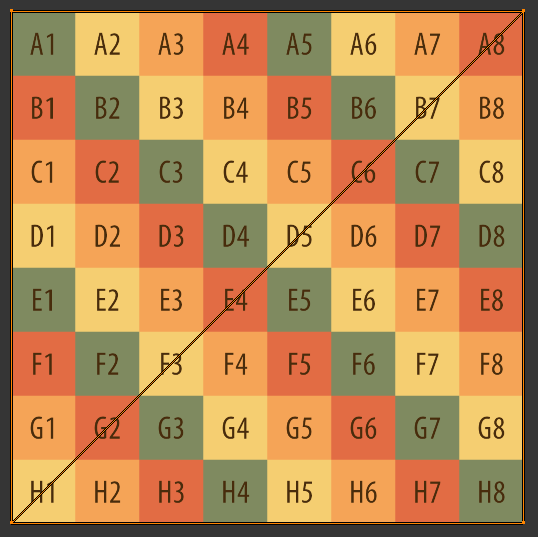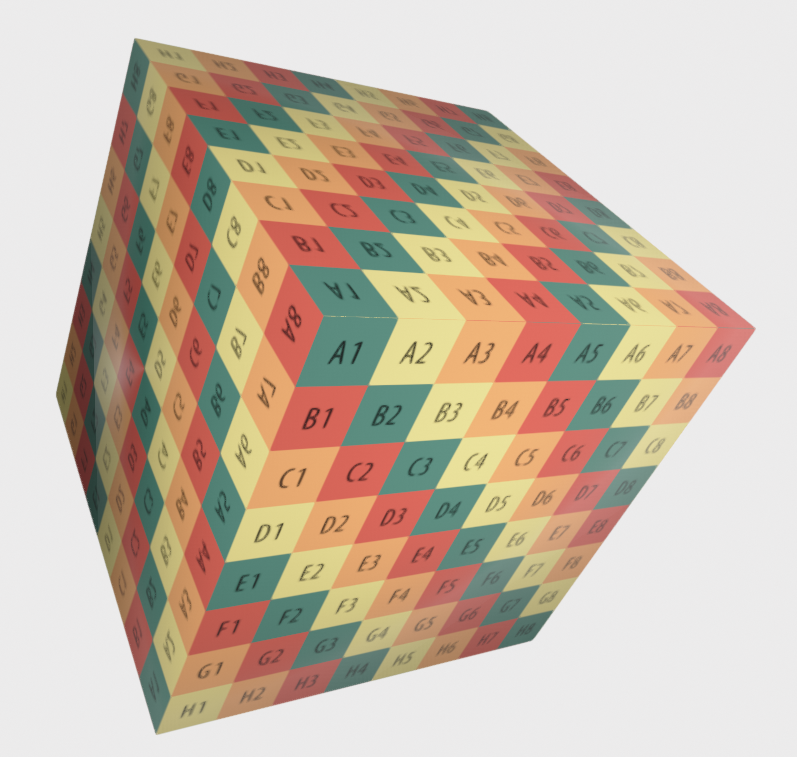### Tetrahedral#

```tetra = o3d.geometry.TriangleMesh.create_tetrahedron(create_uv_map=True)
o3d.visualization.draw({'name': 'tetrahedron', 'geometry': tetra, 'material': material})
```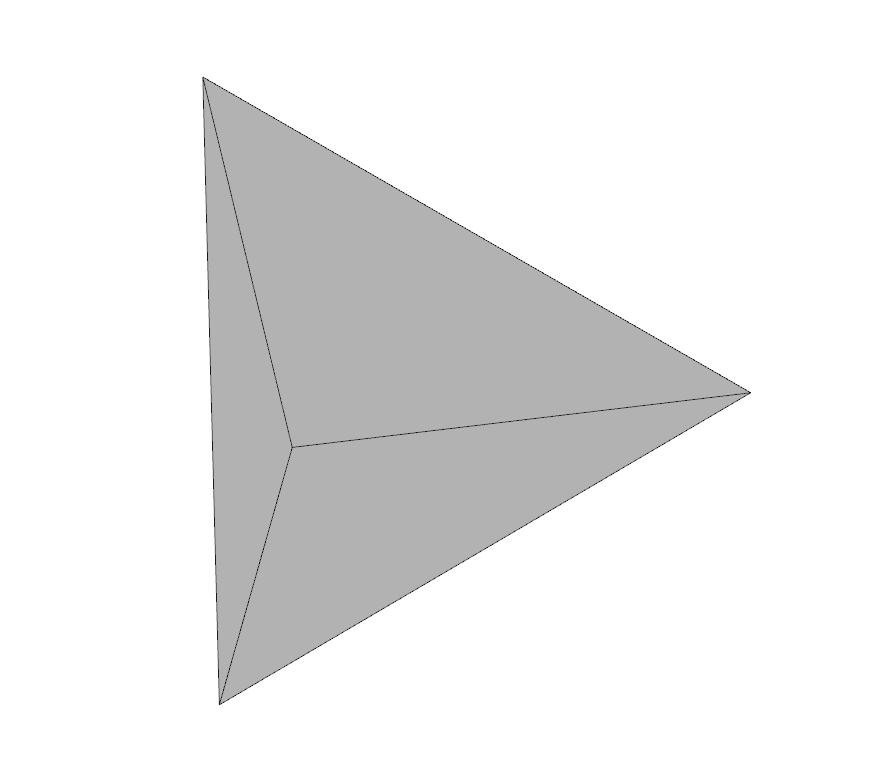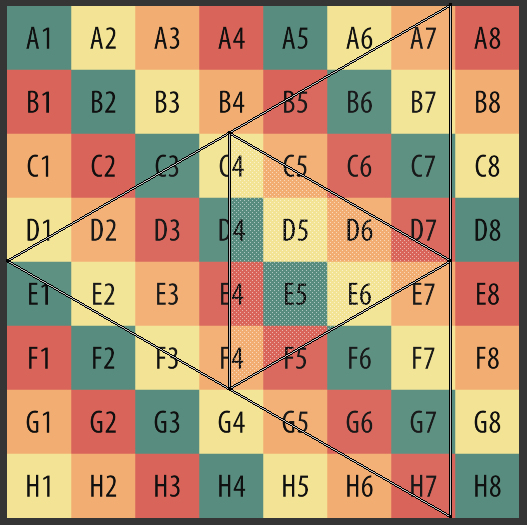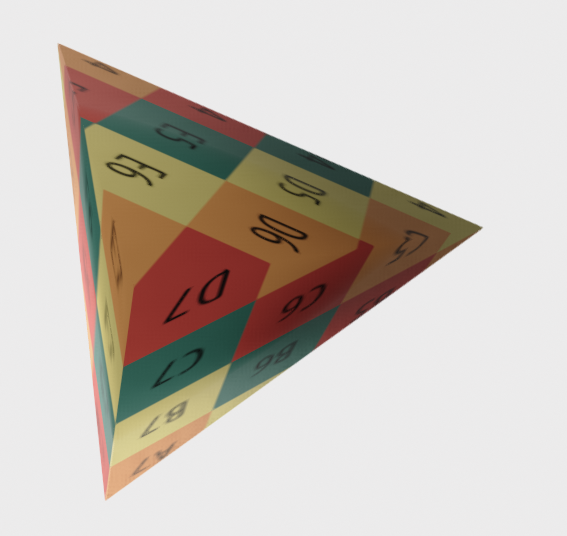### Octahedral#

```octo = o3d.geometry.TriangleMesh.create_octahedron(create_uv_map=True)
o3d.visualization.draw({'name': 'octahedron', 'geometry': octo, 'material': material})
```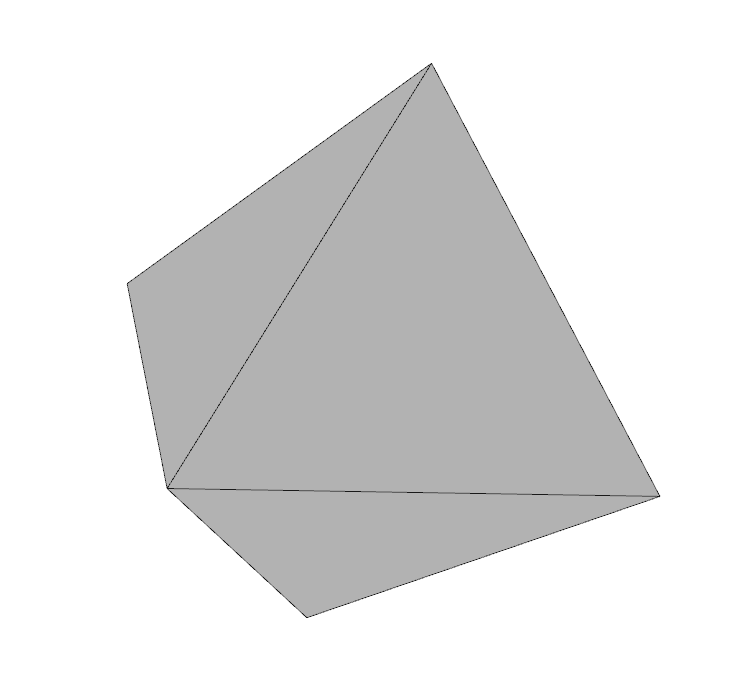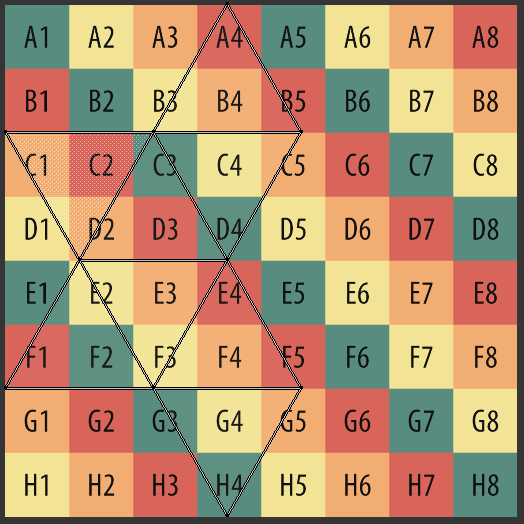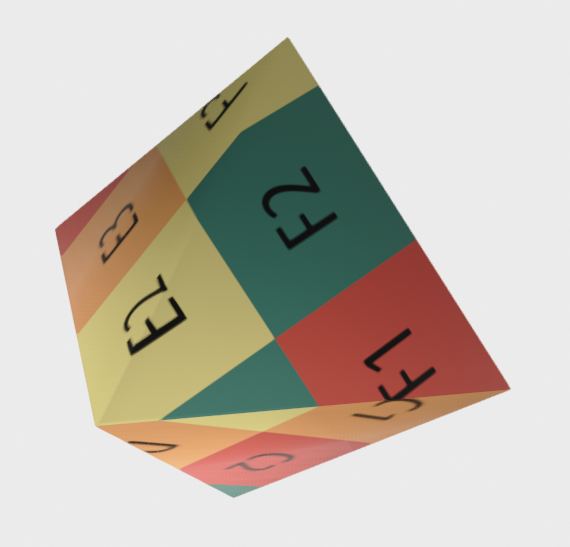### Icosahedron#

```ico = o3d.geometry.TriangleMesh.create_icosahedron(create_uv_map=True)
o3d.visualization.draw({'name': 'icosahedron', 'geometry': ico, 'material': material})
```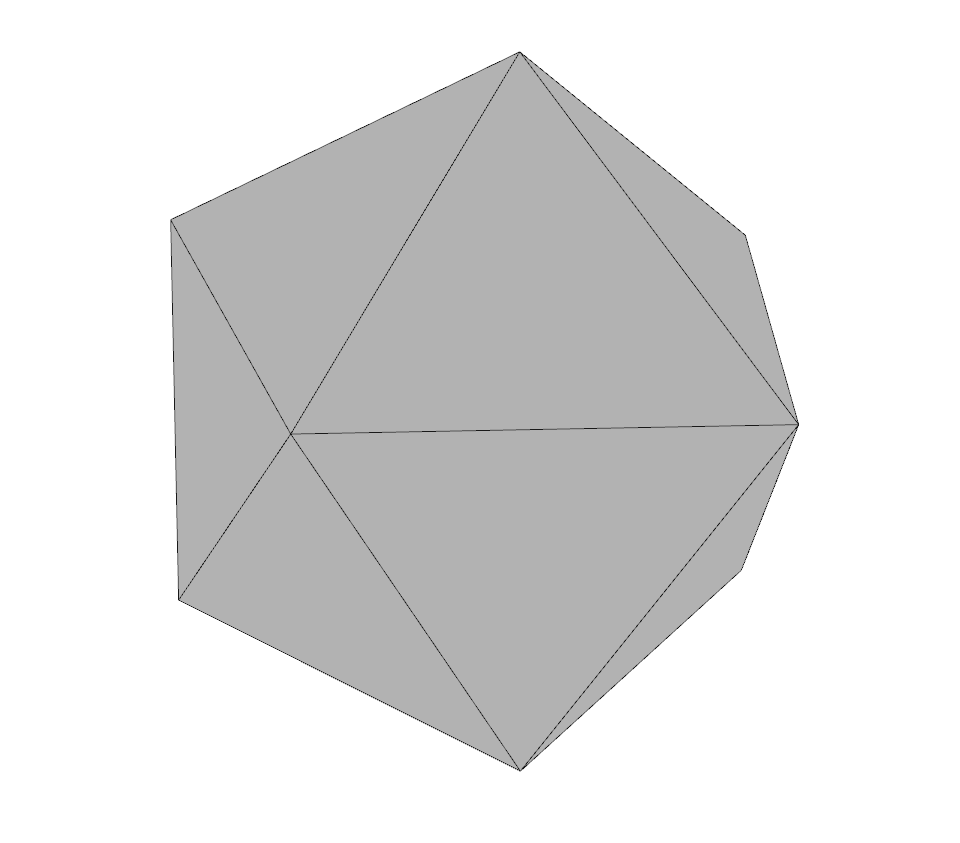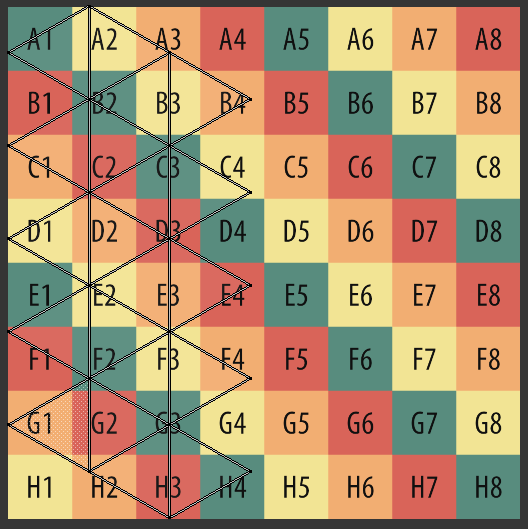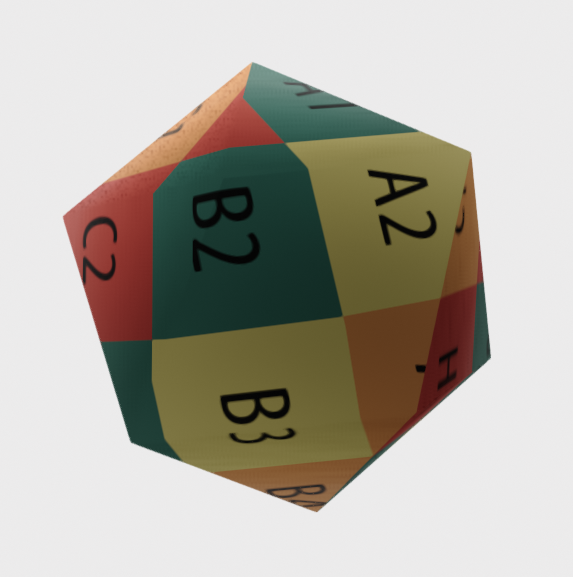### Cylinder#

```cylinder = o3d.geometry.TriangleMesh.create_cylinder(create_uv_map=True)
o3d.visualization.draw({'name': 'cylinder', 'geometry': cylinder, 'material': material})
```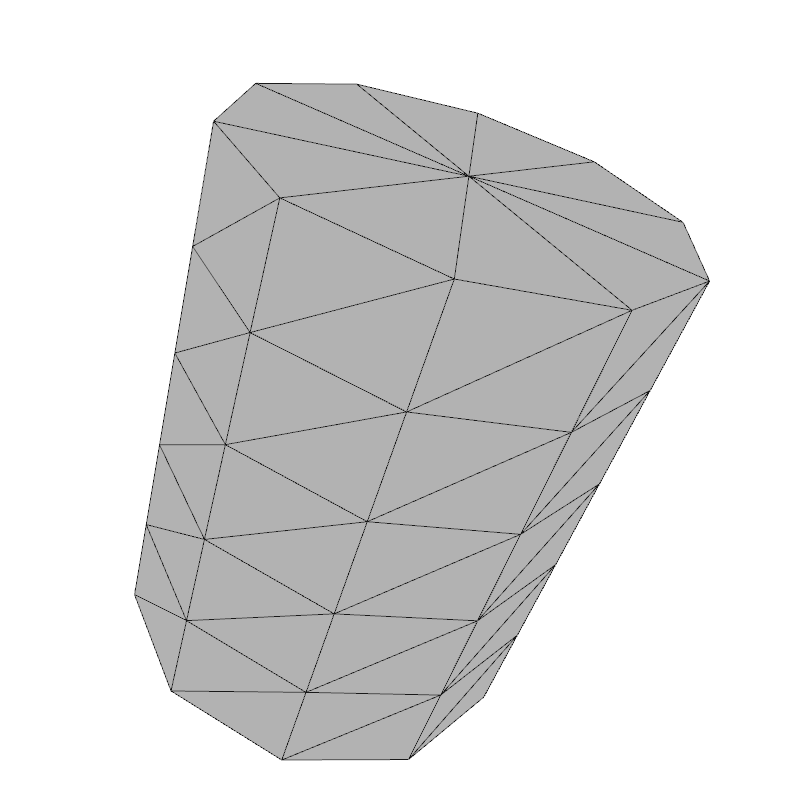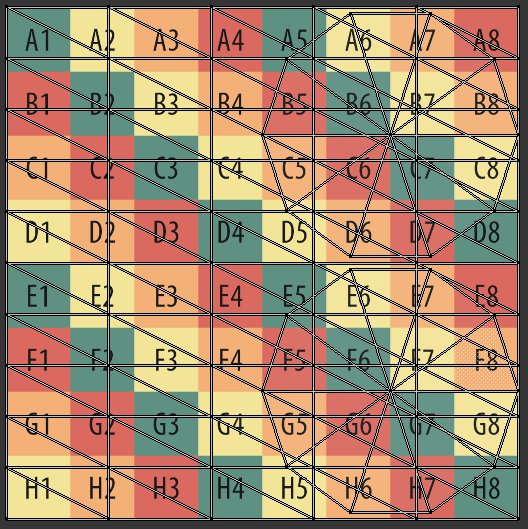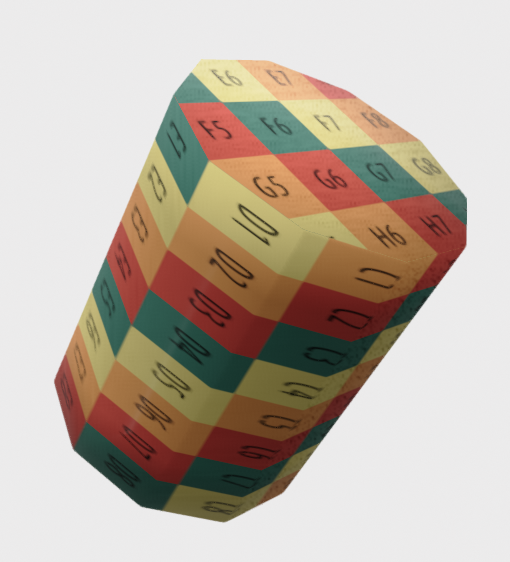### Cone#

```cone = o3d.geometry.TriangleMesh.create_cone(create_uv_map=True)
o3d.visualization.draw({'name': 'cone', 'geometry': cone, 'material': material})
```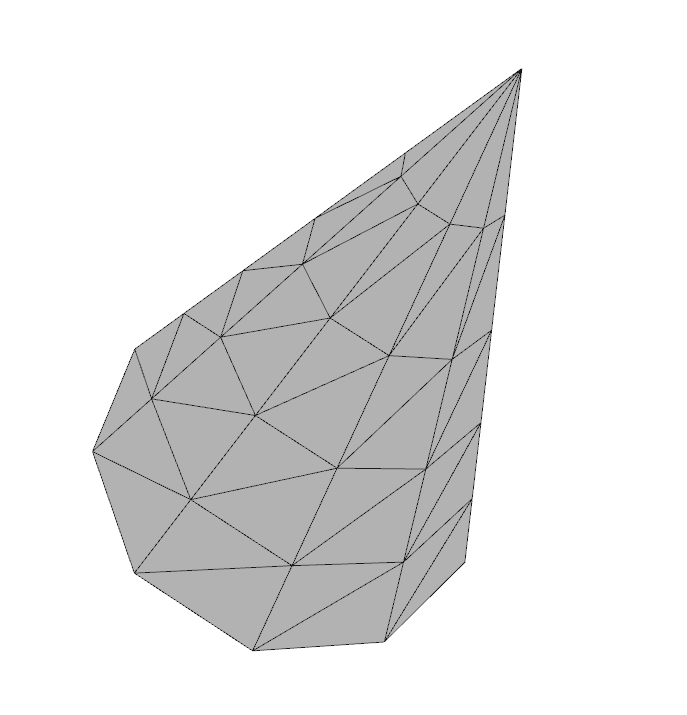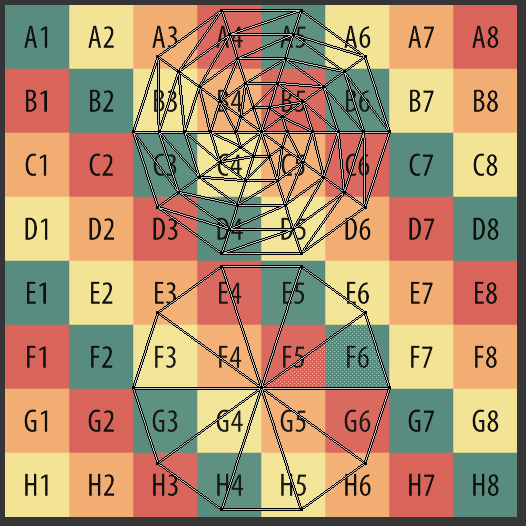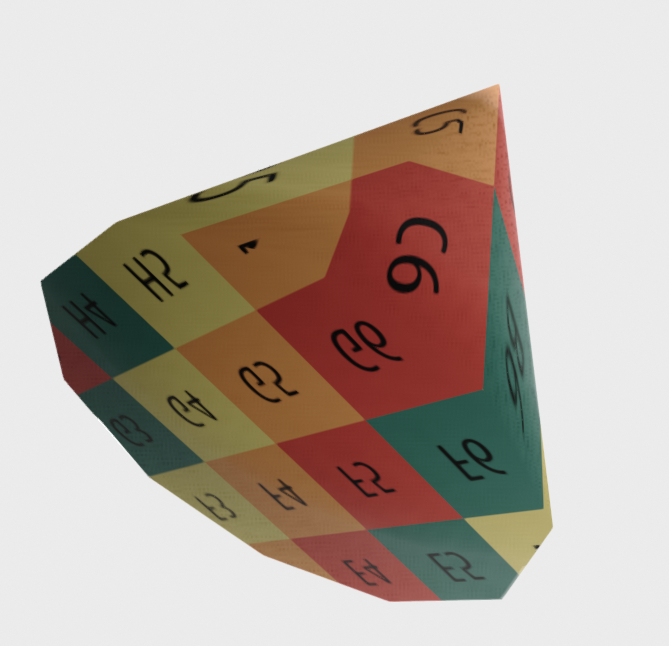### Sphere#

```sphere = o3d.geometry.TriangleMesh.create_sphere(create_uv_map=True)
o3d.visualization.draw({'name': 'sphere', 'geometry': sphere, 'material': material})
```